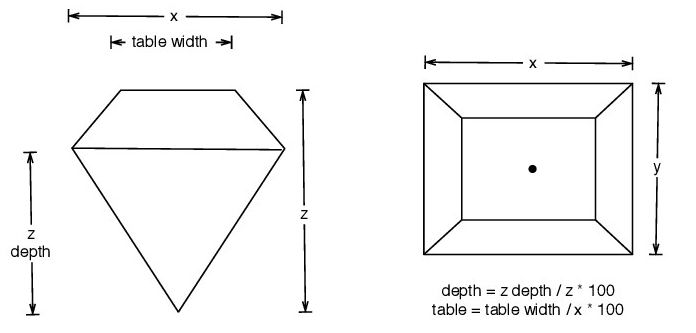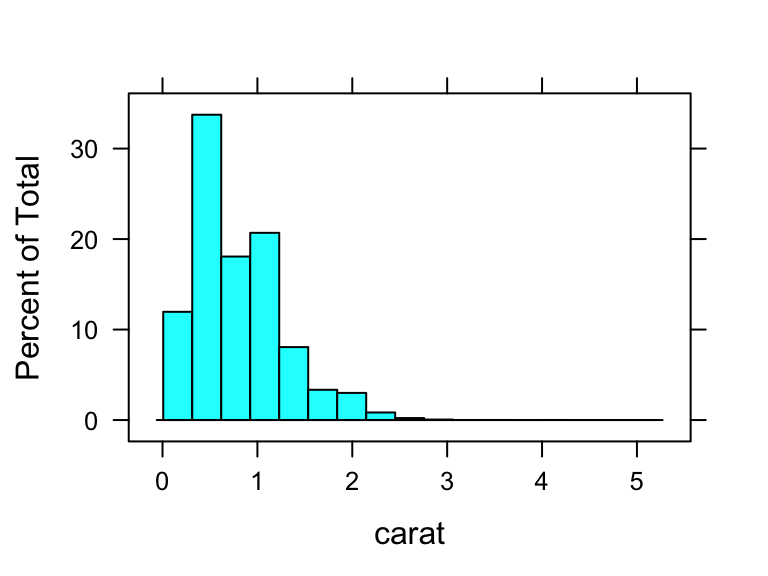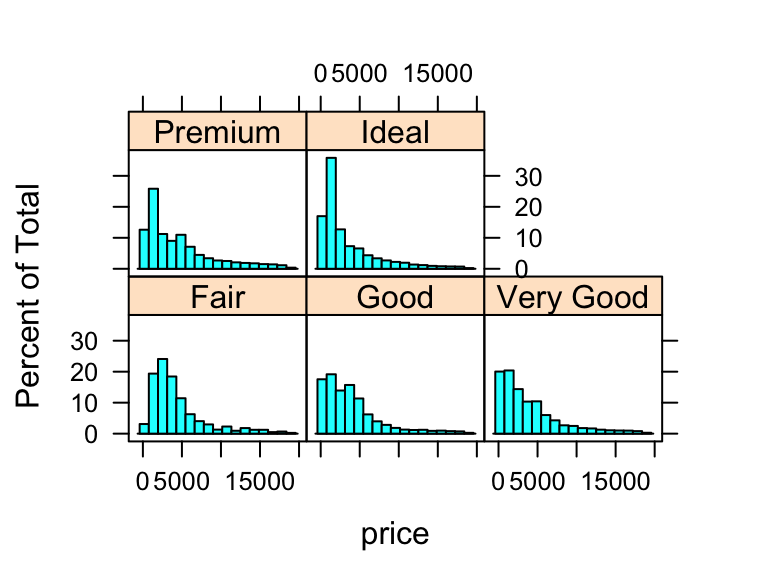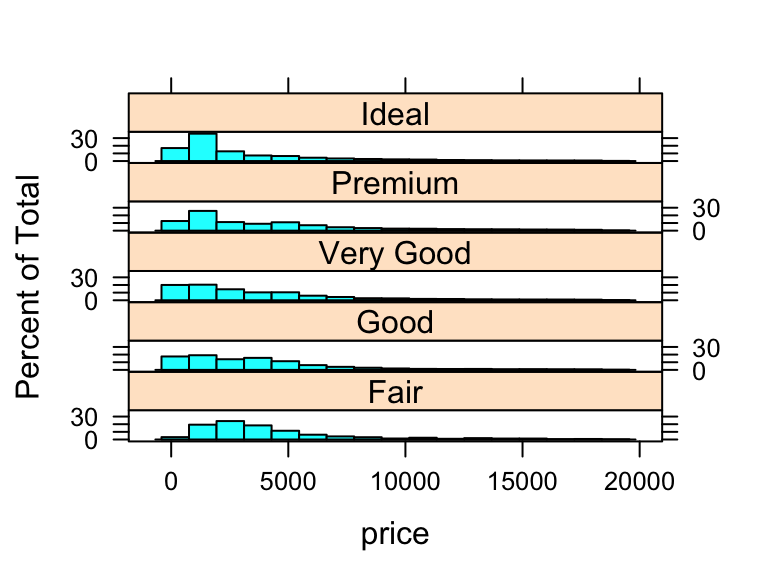Some define Statistics as the field that focuses on turning information into knowledge. The first step in that process is to summarize and describe the raw information - the data. In this lab, we will show you how to use R to get numerical summaries of data and how to view data by graphs. Before we start, we will need to load in the following libraries:

``````library(tidyverse)
library(lattice)``````

## 1. The Diamonds Dataset

The `diamonds` dataset is a built-in data set in the ggplot2 library which is one of the components of the `tidyverse` library, which means that we can access it using the `data()` function after loading the `tidyverse` library

``data(diamonds)``

The variables in the `diamonds` dataset are

• `price`: price in US dollars
• `carat`: weight of the diamond
• `cut`: quality of the cut (`Fair`, `Good`, `Very Good`, `Premium`, Ideal)
• `color`: diamond color, from `J` (worst) to `D` (best)
• `clarity`: a measurement of how clear the diamond is, from `I1` (worst), `SI1`, `SI2`, `VS1`, `VS2`, `VVS1`, `VVS2`, to `IF` (best)

and five physical measurements, `depth`, `table`, `x`, `y` and `z`, as shown in Figure 1 belowFigure 1

The dimension of the `diamonds` dataset is

``dim(diamonds)``
``##  53940    10``

from which we can see the data contains 53940 rows and 10 variables.

To view the names of the variables, type the command

``str(diamonds)``
``````## tibble [53,940 × 10] (S3: tbl_df/tbl/data.frame)
##  \$ carat  : num [1:53940] 0.23 0.21 0.23 0.29 0.31 0.24 0.24 0.26 0.22 0.23 ...
##  \$ cut    : Ord.factor w/ 5 levels "Fair"<"Good"<..: 5 4 2 4 2 3 3 3 1 3 ...
##  \$ color  : Ord.factor w/ 7 levels "D"<"E"<"F"<"G"<..: 2 2 2 6 7 7 6 5 2 5 ...
##  \$ clarity: Ord.factor w/ 8 levels "I1"<"SI2"<"SI1"<..: 2 3 5 4 2 6 7 3 4 5 ...
##  \$ depth  : num [1:53940] 61.5 59.8 56.9 62.4 63.3 62.8 62.3 61.9 65.1 59.4 ...
##  \$ table  : num [1:53940] 55 61 65 58 58 57 57 55 61 61 ...
##  \$ price  : int [1:53940] 326 326 327 334 335 336 336 337 337 338 ...
##  \$ x      : num [1:53940] 3.95 3.89 4.05 4.2 4.34 3.94 3.95 4.07 3.87 4 ...
##  \$ y      : num [1:53940] 3.98 3.84 4.07 4.23 4.35 3.96 3.98 4.11 3.78 4.05 ...
##  \$ z      : num [1:53940] 2.43 2.31 2.31 2.63 2.75 2.48 2.47 2.53 2.49 2.39 ...``````

Here we can see that `carat`, `depth`, `table`, `price` and `x`, `y`, `z` are numerical variables, and `cut`, `color`, and `clarity` are ordinal categorical variables. Specifically, `price` is an integer-valued variable.

## 2. Numerical Summary of Data

To calculate the mean, median, SD, variance, five-number summary, IQR, minimum, maximum of the `price` variable in the `diamonds` dataset, type

``````# Numerical Summary of Data
mean(diamonds\$price)
median(diamonds\$price)
sd(diamonds\$price)
var(diamonds\$price)
fivenum(diamonds\$price)
IQR(diamonds\$price)
min(diamonds\$price)
max(diamonds\$price)``````

In statistics, it is also import to be able to compute averages of variables within specific groupings of the data. For example, in the diamonds dataset the `cut` variable has 5 possible values: [`Fair`, `Good`, `Very Good`, `Premium`, `Ideal`], and we may be interested in the average price of diamonds in each category of cut. To compute this, we can use the `aggregate()` function:

``aggregate(list(price=diamonds\$price), list(cut=diamonds\$cut), FUN=mean)``
``````##         cut    price
## 1      Fair 4358.758
## 2      Good 3928.864
## 3 Very Good 3981.760
## 5     Ideal 3457.542``````

The `aggregate` function requires 3 arguments. The first argument passed to it must be a list containing the columns of data we wish to aggregate. For example, computing a mean, or a sum, or a maximum, are all examples of aggregation. In this case, we want to compute the mean of `price`. The second argument to `aggregate` is a list of variables we wish to group by, which in this case is the `cut` variable. Finally, we must tell the function what aggregation method we wish to use, `FUN=mean` tells the function that we wish to compute a mean. The reason that the second argument is a list is because we may wish to group by multiple variables. For example, try to figure out what the following commands do:

``````aggregate(list(price=diamonds\$price), list(cut=diamonds\$cut, color=diamonds\$color), FUN=median)
aggregate(list(price=diamonds\$price, carat=diamonds\$carat), list(color=diamonds\$color), FUN=min)``````

Surprisingly, higher quality cut diamonds are not necessarily more expensive (e.g., mean price of diamonds with the best cut (Ideal) is \$3457.5, lower than that of the worst cut (Fair), \$4358.7). This is because we didn’t take weight (`carat`) of diamonds into account. Diamonds with Ideal cut tend to be smaller than diamonds with Fair cut.

``````aggregate(list(carat=diamonds\$carat),
list(cut=diamonds\$cut),
FUN=mean)``````
``````##         cut     carat
## 1      Fair 1.0461366
## 2      Good 0.8491847
## 3 Very Good 0.8063814
## 5     Ideal 0.7028370``````

We can find the mean price of diamonds grouped by `cut` and `clarity`:

``````aggregate(list(price=diamonds\$price),
list(cut=diamonds\$cut, clarity=diamonds\$clarity),
FUN=mean)``````

The `aggregate()` function also works for other aggregation methods such as `median()`, `sd()` , `var()` , `min()` , `max()` , `sum()` , `IQR()`.

## 3. Graphical Display of Data

### 3.1 Histogram

Histogram of the `carat` variable:

``histogram(~carat, data=diamonds)``You can adjust the binwidth of the histogram

``histogram(~carat, data=diamonds, width = 0.1)````histogram(~carat, data=diamonds, width = 0.01)``or you can adjust the number of intervals (`nint`)

``````#or you can adjust the number of intervals (nint)
histogram(~carat, data=diamonds, nint = 50)
histogram(~carat, data=diamonds, nint = 500)``````

or you can set the end points of the intervals yourself if you want unequal binwidths

``histogram(~carat, data=diamonds, breaks=c(0.2,0.3,0.5,0.7,1,1.5,2,2.5,3,4,5.5))``

You can split the diamonds by the quality of cut and make separate histograms for each level of `cut`

``histogram(~price | cut, data=diamonds)``You can adjust the number of bins of side-by-side histograms by changing width, nint, or breaks as for a single histogram.

``histogram(~price | cut, data=diamonds, width = 1000)``It’s usually better to stack the five histograms on the same horizontal histogram.

``histogram(~price | cut, data=diamonds, width = 250, layout = c(1,5))``### 3.2 Boxplots

R has a built-in function `boxplot()` for making boxplots, e.g., the boxplot for the price of diamonds

``boxplot(diamonds\$price, horizontal=T)``